In :
import numpy as np
import matplotlib.pyplot as plt
%matplotlib inline
from IPython import display as display
import pandas as pd
import cartopy
import cartopy.crs as ccrs
from cartopy import config
from cartopy.mpl.ticker import LongitudeFormatter, LatitudeFormatter
from cartopy.mpl.gridliner import LONGITUDE_FORMATTER, LATITUDE_FORMATTER
from cartopy import feature as cfeature
from cartopy.feature import NaturalEarthFeature, LAND, COASTLINE, OCEAN, LAKES, BORDERS
import matplotlib.ticker as mticker

from pylab import meshgrid
from matplotlib import cm as cm
import os


### Lecture 27:¶

• Learn how to make an animated gif.

### Animation with Python¶

To truly make animations - for example cartoons - you need some pretty fancy software. There are modules in the Python package mayavi that are pretty powerful, but they are way beyond the scope of this class. On top of that, mayavi is not supported in Python 3, yet, so we can't do it anyway...

However, you CAN make some simple animated gifs with a Python package called imageio (which is not installed by Anaconda). In this class we will work through two examples: one builds on the map of the intensity of the geomagnetic field that we saw before in the lecture on contouring and the second animates the motion of the Indian plate since it rifted off of Africa.

The key to making an animated gif is to generate a series of images that together make the movie. We can already generate figures, so we just need to learn how to stitch them together.

### One hundred years of the Earth's magnetic field¶

In this folder there is a modified version of the magmap function we used in Lecture 21. It now has the map making function embedded in it and will generate a map using the International Geomagnetic Reference Field model for any date between 1900 and 2020.

But before we go on, what IS the IGRF? It is a model for the magnetic field which starts with the premise that magnetic fields (vectors) can be calculated from the gradient of a scalar potential field (${\bf H}=-\nabla \psi_m$). The scalar potential field satisfies Laplace's Equation:

$\nabla^2 \psi_m = 0$.

For the geomagnetic field (ignoring external sources of the magnetic field which are in any case small and transient), the potential equation can be written as:

$\psi_m (r,\theta,\phi)={a\over{ \mu_o} } \sum_{l=1}^\infty \sum_{m=0}^l \left( {a \over r}\right)^{l+1} P_l^m (\cos \theta) \left(g_l^m \cos m\phi + h_l^m \sin m\phi\right),$

where $a$ is the radius of the Earth ($6.371$ x $10^6$ m). In addition to the radial distance $r$ and the angle away from the pole $\theta$, there is $\phi$, the angle around the equator from some reference, say, the Greenwich meridian. $\theta$ is the co-latitude and $\phi$ is the longitude. The $g_l^m$s and $h_l^m$s are the gauss coefficients (degree $l$ and order $m$) for hypothetical sources at radii less than $a$ calculated for a particular year. These are normally given in units of nanotesla. The $P_l^m$s are wiggly functions called partially normalized Schmidt polynomials of the argument $\cos \theta$. These are closely related to the associated Legendre polynomials. The first few of $P_l^m$s are: $$P_1^0=\cos \theta, P_2^0 = {1\over 2}(3\cos^2\theta -1), \hbox{ and } P_3^0 = {1\over 2}\cos \theta(5\cos^3\theta -3\cos \theta ),$$

which we can plot here:

In :
P1 = lambda theta: np.cos(theta)
P2= lambda theta: 0.5*(3.*np.cos(theta)**2-1.)
P3 = lambda theta: 0.5*np.cos(theta)*(5.*np.cos(theta)**3-3.*np.cos(theta))

thetas=np.radians(np.arange(0,180)) # make an array of thetas from 0 to pi
P1s=P1(thetas) # evaluate the first Legendre polynomial
P2s=P2(thetas) #second
P3s=P3(thetas) # third
plt.plot(thetas,P1s,'r-',label='P$_1$') # plot the first
plt.plot(thetas,P2s,'b-',label='P$_2$')
plt.plot(thetas,P3s,'g--',label='P$_3$')
plt.xlabel('$\theta$') # label X axis
plt.ylabel('Legendre Polynomials') # label Y
plt.legend(loc=3); # put on a legend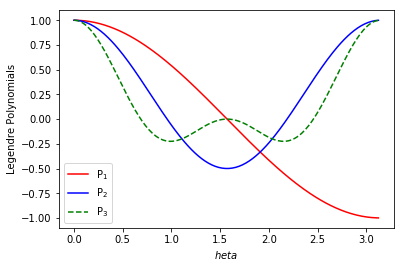So, like the Fourier transform we learned about in the last lecture, these functions could be used to make a 'spherical harmonic analysis' of spherical data like the Earth's magnetic field. From observations of the magnetic field around the Earth, we can fit these equations with the Gauss Coefficients and use the potential equation to predict magnetic field vectors anywhere outside the source (the outer core).

New lists are compiled every five years or so and you can download them from here: https://www.ngdc.noaa.gov/IAGA/vmod/igrf.html I downloaded IGRF12 file and put it into a module called mkigrf along with functions to do the calculations.

OK. Let's work through this script first:

In :
import mkigrf  # import the module

def magmap2png(date,lon_0,element):
"""
Generates a map of the desired geomagnetic element.
Inputs:
date: decimal year
lon_0: central longitude for the map
element: desired field element for the map
Allowed elements are:
D (declination),
I (inclination),
B (total field strength), and
Output:
Hammer projection of desired element saved  to a .jpg file with the field element
and date in the file name.
"""
incr=5 # this sets the lat/lon grid size of the field model in degrees
# adjust geographic bounds for the desired resolution.
# Remember that % is for the modulo and makes sure that the longitudes are 0-360 range
lonmax=(lon_0+180.)%360+incr # maximum longitude for the array
lonmin=(lon_0-180.) # minimum longitude
latmax=90+incr
lons=np.arange(lonmin,lonmax,incr) # makes an array of longitudes
lats=np.arange(-90,latmax,incr) # same for latitudes
# sets up some containers we might want
B=np.zeros((len(lats),len(lons)))  # 2D array of lats and lons
Binc=np.zeros((len(lats),len(lons)))
Bdec=np.zeros((len(lats),len(lons)))
for j in range(len(lats)): # steps through all the latitudes
for i in range(len(lons)): # and the longitudes
x,y,z,f=mkigrf.doigrf(lons[i],lats[j],date) # evaluates the field at place and time
Dec,Inc,Int=mkigrf.cart2dir(x,y,z) # converts to declination, inc, strength
B[j][i]=Int*1e-3 # converts from nT to microtesla
Binc[j][i]=Inc
Bdec[j][i]=Dec
Brad[j][i]=z*1e-3 # converts from nT to microtesla
# make the map
# assigns array mag to desired element
if element=='B':
mag=np.array(B)
title='Field strength ($\mu$T) '
elif element=='D':
mag=np.array(Bdec)
title='Declination '
elif element=='I':
mag=np.array(Binc)
title='Inclination '
elif element=='R':
plt.clf() # clears the plot in case you call it multiple times
ax = plt.axes(projection=ccrs.Mollweide(central_longitude=lon_0))
x, y = np.meshgrid(lons,lats)
m=ax.contourf(x, y, mag, levels=np.arange(20,75,5),transform=ccrs.PlateCarree(),cmap=cm.RdYlBu)
ax.set_global()
ax.coastlines()
cbar=plt.colorbar(m,orientation='horizontal') # put on a color bar of intensities
plt.title('Field strength ($\mu$T): '+str(date));
if element=='B':title='Intensity' # renames the title to take out spaces
plt.savefig('Maps/'+title.strip()+'_'+str(date)+'.png') # saves the  figure. to a folder


Now we need a little for loop to generate maps for the desired element and date range. But first, we need a place to put them. We can tell if that directory already exists by getting a listing of our working directory. If it doesn't we can make a directory called 'Maps'. Then we can clear it out, in case there are any maps left over from a previous attmept.

In :
L=os.listdir("./")
if 'Maps' not in L:
os.mkdir('Maps')
!rm Maps/*.png


And now we can make a bunch of maps. Warning: it takes a while to run this.

In :
dates=range(1900,2018,10) # make maps for these years.
lon_0=0 # center the maps at the Greenwich meridian
element='B' # let's do field strength
for date in dates:  # step through the loop
magmap2png(date,lon_0,element) # make the maps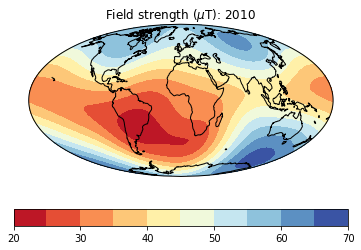Once we've made all the maps, we can put them into an animation using a module called imageio. To use imageio, you will have to first install it. The most convenient way to do this is using 'pip install update imageio'. Uncomment that line if you need to install it.

In :
#!pip install update imageio
import imageio # and let's go ahead and import it.


And now we can run it.

We can use the function os.listdir to get a list of files in the 'Maps' directory. Then work through the files with the imageio.imread() function. Then save the collected images to an animated gif file. :)

In :
filenames=os.listdir('Maps/') # listing of the directory
images = [] # make a container to put the image files in
for file in filenames: # step through all the maps
if '.png' in file: # skip some of the nasty hidden files
filename='Maps/'+file # make filename from the folder name and the file name
imageio.mimsave('Maps/Bmovie.gif', images) # save to an animated gif.


To watch the video, just choose 'Open' from the File menu, find the gif and open it. :)

OR, to embed it in a notebook, here's a handy trick:

In :
from ipywidgets import Image as Image # don't overwrite Image
animatedGif = "Maps/Bmovie.gif" #path relative to your notebook
file = open(animatedGif , "rb")
progress= Image(
value=image,
format='gif')
display.display(progress)


### Continental Drift - the movie¶

The idea of continental drift, controversial for the first half of the 20$^{th}$ century, was basically proven in the 50s by the concept of apparent polar wander. So what is apparent polar wander? By assuming that the magnetic field is generated by a magnetic dipole at the center of the Earth and that it is constrained to be aligned with the spin axis, it is possible to determine the position of the spin axis through time. In the 50s it was recognized that this pole seemed to move with respect to the spin axis, so this apparent wandering could be interpreted in two ways: wandering of continents whose paleomagnetic directions reflect the changing orientations and distances to the (fixed) pole (lefthand figure below), or alternatively, the pole itself could be wandering, as in righthand figure while the continent remains fixed.

In :
display.Image(filename='Figures/wandering.png',width=500)

Out: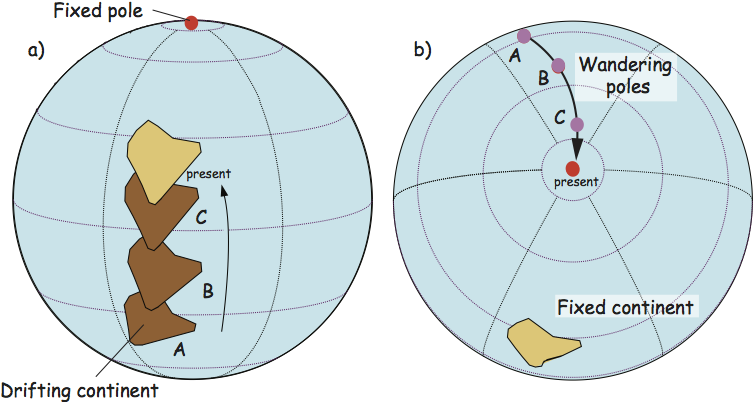a) A moving continent will retain a record of changing paleomagnetic directions through time that reflect the changing orientations and distances to the pole (which is held fixed). The resulting path of observed pole positions is called an "apparent polar wander path" or APWP because in this case the pole is actually fixed and only appears to move when viewed from the continental frame of reference. b) On the other hand, if a continent is held fixed, the same changing paleomagnetic directions reflect the wandering of the pole itself. This is called "true polar wander" or TPW. [Figure from Essentials of Paleomagnetism by Tauxe et al.,(2010): https://earthref.org/MagIC/books/Tauxe/Essentials/]

Data from a single continent cannot distinguish between these two hypotheses. But data from multiple continents and a firm belief in the essential dipolar nature of the geomagnetic field (dating back to 1600!) can. If the pole paths from two or more continents diverge back in time and there is a dipolar field (only one north pole), then it must be the continents that are doing the wandering. It was data of this kind that convinced paleomagnetists in the 50s of the reality of continental drift.

Well after the concept of continental drift and apparent polar wander had been accepted by most of the paleomagnetic community, the idea of sea-floor spreading and plate tectonics was developed to explain it. In plate tectonics, the hard outer shell of the Earth, the lithosphere is composed of many rigid or semi-rigid plates. These plates are in constant motion with respect to one another. The relative motion of two plates can be described by rotation about an Euler rotation vector, which is usually specified by a pole latitude/longitude on the surface of the Earth ($\lambda_{e},\phi_e$) and a rotation rate $\omega$ in $^{\circ}$Myr$^{-1}$. The velocity $v$ at a given point on a plate with respect to its "fixed" partner varies as a function of angular distance from the Euler pole ($\theta$) as:

$v = a \omega \sin \theta,$

where $a$ is the radius of the Earth. As an example, the figure below shows the motion of North America (NAM) with respect to "fixed" Europe (EUR). The current Euler pole is the square. Lines of co-latitude correspond to $\theta$ in this projection, so the velocities (usually expressed in cm/yr; see black arrows in the right hand figure increase away from the pole, with a maximum at $\theta = 90^{\circ}$. Beyond 90$^{\circ}$ the velocities decrease to the antipode of the Euler pole. Spreading rates can be determined from marine magnetic anomalies and their variation along the ridge crest can be fit with the spreading rate equation to find both $\omega$ and $\theta$, and helping to constrain the location of the Euler pole.

In :
display.Image(filename='Figures/plates.png')

Out: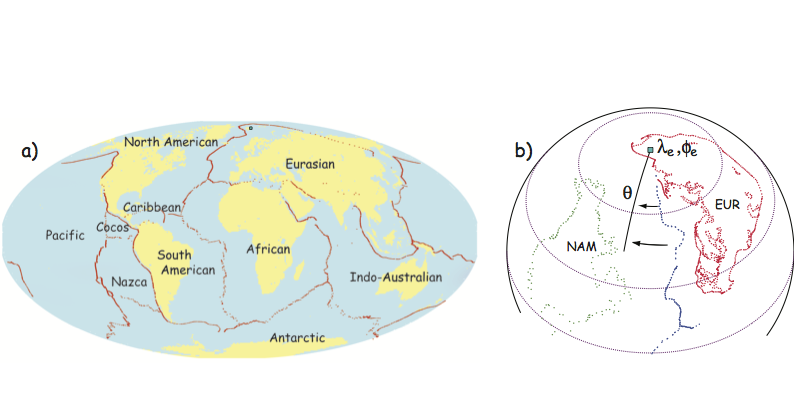a) Some of the major lithospheric plates. b) Motion of North America with respect to Europe around the Euler pole shown as a blue square. Projection is such that current Euler pole North America (NAM) with respect to Europe (EUR) is at the "North pole". Lines of co-latitude are the angular distance from the Euler pole, $\theta$. Velocities of NAM with respect to EUR at two points with different $\theta$ are shown as black arrows. [Figure from Essentials of Paleomagnetism by Tauxe et al.,(2010): https://earthref.org/MagIC/books/Tauxe/Essentials/]

### Euler rotations and finite rotations¶

Euler poles describe instantaneous rates of rotation of one plate or continental fragment with respect to another. Often, what is known is not the rate, but the total rotation about a given pole that restores a plate to some prior state. Such a pole is called a finite rotation pole.

In :
display.Image(filename='Figures/finrot.png',width=300)

Out: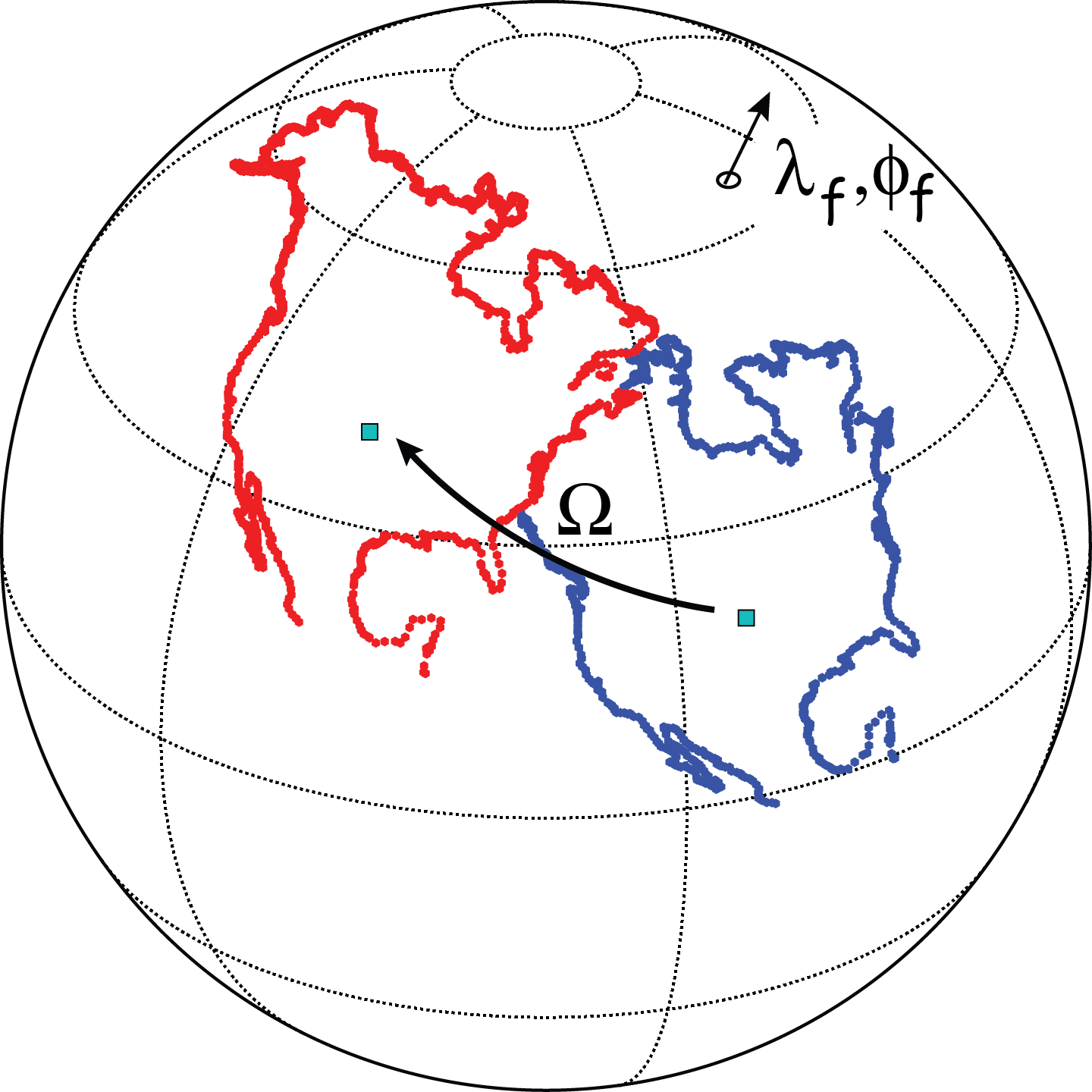Finite rotation of North America from one frame of reference to another. Finite rotation pole is located at $\lambda_f,\phi_f$ and the finite rotation is $\Omega$. [Figure from Essentials of Paleomagnetism by Tauxe et al.,(2010): https://earthref.org/MagIC/books/Tauxe/Essentials/]

A huge amount of work has been put into compiling finite rotations for various lithospheric plates through time. Using these poles of rotation, the points defining a particular continental fragment can be rotated back through time as was done for the picture of India moving north above, which is in fact a series of reconstructions of the Indian subcontinent consistent with paleomagnetic poles determined at intervals for the last 80 Ma. Now we can make the movie version.

All we need are the continental outlines (which I put into the module continents) and the finite rotations which I put into a module called poleRot for your rotation pleasure. There is a directory called ContRot for storing the images in (just like we did for the magnetic field maps.

So to get started, a little housekeeping:

In :
!rm ContRot/*.jpg
import poleRot
help(poleRot)

rm: ContRot/*.jpg: No such file or directory
Help on module poleRot:

NAME
poleRot

FUNCTIONS
PTrot(EP, Lats, Lons)
Does rotation of points on a globe  by finite rotations, using method of Cox and Hart 1986, box 7-3.

cart2dir(cart)
Converts a direction in cartesian coordinates into declination, inclinations

Parameters
----------
cart : input list of [x,y,z]

Returns
-------
direction_array : returns an array of [declination, inclination, intensity]

dir2cart(d)
Converts a list or array of vector directions in degrees (declination,
inclination) to an array of the direction in cartesian coordinates (x,y,z)

Parameters
----------
d : list or array of [dec,inc] or [dec,inc,intensity]

Returns
-------
cart : array of [x,y,z]

get_continent(continent)
Returns the continental outlines (latitudes and longitudes) for
these continents:
af=Africa
aus=Australia
balt=Baltica
congo=Congo
eur=Europe
ind=India
sam=South America
ant=Antarctica
grn=Greenland
lau=Laurentia
nam=North America
waf=West Africa
gond=Gondwana
kala=Kalahari

get_pole(continent, age)
Returns the finite pole of rotation for a given continent and age
Pole rotations from Torsvik (2008).

FILE
/Users/ltauxe/Dropbox/4SIO113_2019/4GIT/poleRot.py



We can make the map of Africa (assumed fixed) and India in their present positions by retrieving the continental outlines from the continents module and plotting them using the tricks learned in Lecture 18.

Let's make this a function so we can call it with whatever continental outline and color we choose:

In :
def cont_plot(lats,lons,color):
ax = plt.axes(projection=ccrs.Orthographic(40, 20))
gl=ax.gridlines(crs=ccrs.PlateCarree(),color='black',linewidth=1,linestyle='dotted')
gl.ylocator=mticker.FixedLocator(np.arange(-80,81,20))
gl.xlocator=mticker.FixedLocator(np.arange(-180,181,60));
gl.xformatter = LONGITUDE_FORMATTER
gl.yformatter = LATITUDE_FORMATTER
ax.plot(lons,lats,color=color,transform=ccrs.Geodetic())
ax.set_global();
af_data=np.array(poleRot.get_continent('af')).transpose()
lats=af_data  # fish out the latitudes
lons=af_data # fish out the longitudes
cont_plot(lats,lons,'blue')
## then do the same for India
ind_data=np.array(poleRot.get_continent('ind')).transpose()
lats=ind_data
lons=ind_data
cont_plot(lats,lons,'red')

#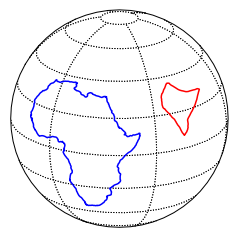Now we can step through time to make a series of images as India peels off from Africa and moves north:

In :
Ages=range(110,0,-5) # do 10 Ma steps
# step through ages for movie
cnt=0 # this is a counter for the figure names.
af_data=np.array(poleRot.get_continent('af')).transpose()
aflats=af_data  # fish out the latitudes
aflons=af_data # fish out the longitudes
for age in Ages:
plt.clf() # each time we plot a map we need to clear off the old one.
cont_plot(aflats,aflons,'blue')
# get the pole of rotation for india at the desired age
pole=poleRot.get_pole('ind',age)
rotlats,rotlons=poleRot.PTrot(pole,ind_data,ind_data) # do the rotation
cont_plot(rotlats,rotlons,'red')
num=str(cnt) # make a string of the figure number
cnt+=1 # increment that
while len(num)<3:num='0'+num # pad the figure number with 00's
plt.title(str(age)+' Ma') # put on  a title
plt.savefig('ContRot/contRot_'+num+'.png') # save the figure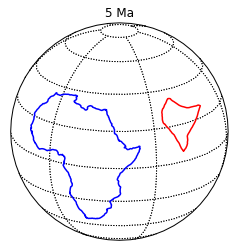In :
filenames=os.listdir('ContRot/') # listing of the directory
images = [] # make a container to put the image files in
for file in filenames: # step through all the maps
if '.png' in file: # skip some of the nasty hidden files
filename='ContRot/'+file # make filename from the folder name and the file name
imageio.mimsave('ContRot/ContRot.gif', images) # save to an animated gif.


And, as before, you can open the animated gif ContRot.gif under the File menu. Or, embed it in this notebook:

In :
animatedGif = "ContRot/ContRot.gif" #path relative to your notebook
file = open(animatedGif , "rb")